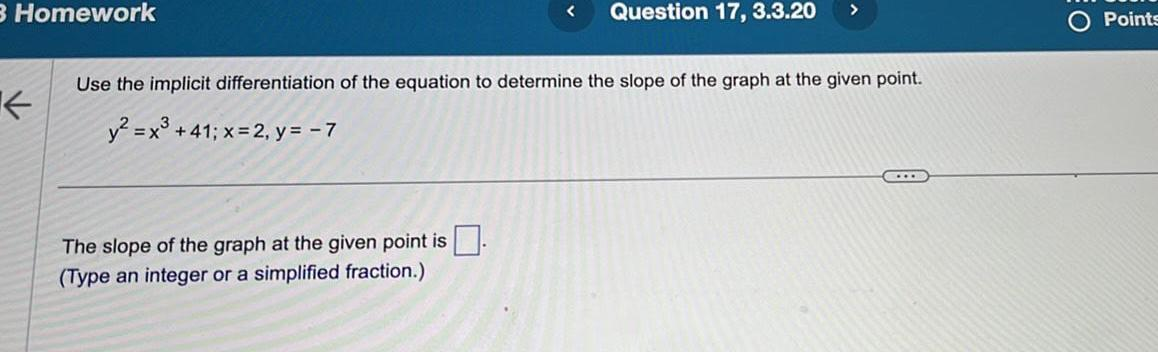Question:

# 3 Homework K Question 17 3 3 20 Use the implicit

Last updated: 9/3/20233 Homework K Question 17 3 3 20 Use the implicit differentiation of the equation to determine the slope of the graph at the given point y x 41 x 2 y 7 The slope of the graph at the given point is Type an integer or a simplified fraction Points